# Tutorial: How To Subtract Dates In Redshift

In this post, we’ll walk through three ways to subtract dates (or timestamps) in Redshift:

1. subtracting time intervals from dates
2. subtracting integers (as days) from dates

Note: The example queries/syntax are the same in Postgres, and are similar (if not the same) in other "flavors" of SQL.

## Prerequisite knowledge: Getting current date/time values

If you already know how to get the current date/time, feel free to skip this section. If not, this should make a difference for you (see what I did there?)

`SELECT CURRENT_DATE` will give you the current date as Date data type (yyyy-mm-dd 00:00:00). `SELECT CURRENT_TIMESTAMP` will give you the current date/time information as a Timestamp data type (yyyy-mm-dd hh:mm:ss) in “time zone” UTC. You can change this value to Eastern time by changing the query to `SELECT CURRENT_TIMESTAMP at time zone 'est'`. You can learn more about Dates and Timestamps here.

Now that you can get the current date/time, let’s subtract some dates!

## Here are 3 ways to get yesterday’s date

#### Subtract an interval from a date

`SELECT CURRENT_DATE - interval '1 day' AS yesterday;`

#### Output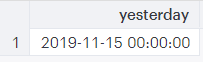#### Subtract an integer from a date

`SELECT CURRENT_DATE - 1 AS yesterday;`

#### Output`SELECT DATEADD('day', -1, CURRENT_DATE) AS yesterday;`

#### Output## Subtracting Intervals From Dates

An interval is a data type that represents a period of time between two datetime values. Interval syntax is expressed as the keyword “interval”, followed by a string (contained in single quotes) with a number value and a unit. Units can be: microsecond, millisecond, second, minute, hour, day, week, month, year, decade, century, millennium (including plural versions of each unit and abbreviations). Intervals can be positive or negative. For example, ‘1 week’, ‘-1 week’, ‘2.5 mon’, and ‘5 years’ are all intervals. Intervals can combine time units. Interval ‘1 week 3 days’ is equal to interval ‘10 days’. You can also multiply an integer by an interval, like 28 * ‘1 day’.

#### Get previous week (by subtracting an interval)

```SELECT   CURRENT_DATE AS date,
CURRENT_DATE - interval '1 week' AS one_week_ago;```

#### Output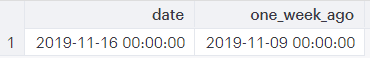This query takes the current date and inserts the value in a column called “date”. It subtracts the time interval ‘1 week’ from the current date and puts the result in a column called “one_week_ago”. Since CURRENT_DATE is a Date value, the result is also a Date value.

## Subtracting Integers From Dates

You can subtract an integer from a Date or Timestamp without an interval unit because the time unit in Dates and Timestamps is days.

#### Get previous day (by subtracting an integer)

```SELECT
CURRENT_DATE AS t1,
CURRENT_DATE - 1 AS t2,
t1 - t2 AS date_difference;```

#### Output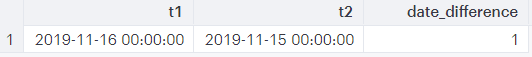This query takes the current date value and puts it in a column labeled “t1”. It takes the current date minus 1 (day) and puts the result in a column labeled “t2”. The last column is the difference between “t1” and “t2”, and is labeled “date_difference”. You can see that the difference between the value in “t1” and “t2” is 1 day.

#### Get previous day/time (by subtracting an integer)

```SELECT
CURRENT_TIMESTAMP AS t1,
CURRENT_TIMESTAMP -1 AS t2,
t1 - t2 AS date_difference;```

#### Output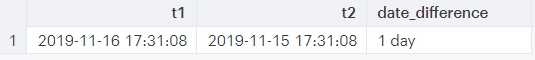This query is the same as the previous one except we used CURRENT_TIMESTAMP instead of CURRENT_DATE. “Date_difference” (“t1” - “t2”) is 1 day in both cases.

## Using DATEADD() to Subtract Dates

#### Get previous year (using DATEADD())

```SELECT
CURRENT_DATE AS today,

#### Output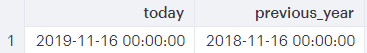The DATEADD() function has 3 parts: time unit, number (this is the number of time units — in this case ‘years’ — that will be added to the start date), start date(or date/time). This query adds -1 years to the current date. The result is displayed in a column called “previous_year”.

As you can see, there are many ways to get to the same result. In fact, the following query demonstrates SEVEN ways you could find “2 days ago”. Pretty cool.

```SELECT
CURRENT_DATE AS date,
CURRENT_DATE - 2,
CURRENT_DATE - interval '2 days',
CURRENT_DATE - interval '2 day',
CURRENT_DATE + interval '2 days ago',
CURRENT_DATE + interval '-2 day',
CURRENT_DATE - 2 * interval '1 day',
CURRENT_DATE + -2 * interval '1 day';```

## Up next

Now that you’re an expert at subtracting (and adding) dates and timestamps in SQL, stay tuned for our next post where we will cover finding the difference between two dates more in depth. Thanks for reading this post and for learning SQL with Panoply. As always, we are open to feedback and would love to hear what you’d like to learn more about. Contact us here.Get a free consultation with a data architect to see how to build a data warehouse in minutes.